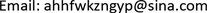1. 引言

2. 系统原理构造

3. 系统计算机仿真和理论分析3.1. 电动空调分析

Q = k A Δ t (1)

3.2. 管道及其控制系统分析

W 势 能 + W 动 能 + W 内 能 + W 压 强 能 = 固 定 值 (2)

x ∘ = f ( x , p , v , t ) , (3)

x ( t 0 ) = x 0 , (4)

W ( x ( t g ) , t g ) = 固 定 值 . (5)

3.3. 电池箱体仿真和分析

K A ( X a − X ) = c m d X d t (6)

∫ t t ξ c ρ V 1 ( X a − 10 ) d t = c ρ V 2 d X a d t + W 损 耗 (7)

φ = φ ( X a ( t ) , X ( t ) , t ) ; (8)

3.4. 表冷器仿真和分析

c 水 M a ( T w o − T w i ) = c 气 M a ( T a o − T a i ) ; (9)

M = ρ S υ t ; (10)

4. 水循环恒温系统实体实验

6. 结语